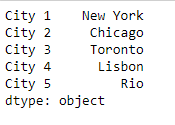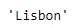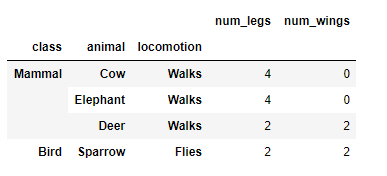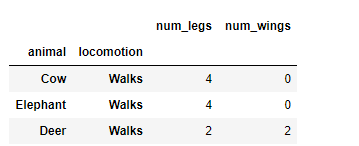Open in App
Not now

# Python | Pandas Series.xs

• Last Updated : 28 Jan, 2019

Python is a great language for doing data analysis, primarily because of the fantastic ecosystem of data-centric python packages. Pandas is one of those packages and makes importing and analyzing data much easier.

Pandas series is a One-dimensional ndarray with axis labels. The labels need not be unique but must be a hashable type. The object supports both integer- and label-based indexing and provides a host of methods for performing operations involving the index.

Pandas` Series.xs()` function return a cross-section from the Series/DataFrame for the given key value.

Syntax:Series.xs(key, axis=0, level=None, drop_level=True)

Parameters :
key : Label contained in the index, or partially in a MultiIndex.
axis : Axis to retrieve cross-section on.
level : In case of a key partially contained in a MultiIndex, indicate which levels are used. Levels can be referred by label or position.
drop_level : If False, returns object with same levels as self.

Returns : Series or DataFrame

Example #1: Use `Series.xs()` function to return a cross-section of the given Series object for the passed key value.

 `# importing pandas as pd``import` `pandas as pd`` ` `# Creating the Series``sr ``=` `pd.Series([``'New York'``, ``'Chicago'``, ``'Toronto'``, ``'Lisbon'``, ``'Rio'``])`` ` `# Creating the row axis labels``sr.index ``=` `[``'City 1'``, ``'City 2'``, ``'City 3'``, ``'City 4'``, ``'City 5'``] `` ` `# Print the series``print``(sr)`

Output :Now we will use `Series.xs()` function to return the cross-section for the given series object.

 `# return cross-section corresponding to``# the 'City 4' label``sr.xs(key ``=` `'City 4'``)`

Output :As we can see in the output, the `Series.xs()` function has returned ‘Lisbon’ as the cross-section for the given Series object.

Example #2 : Use `Dataframe.xs()` function to return a cross-section of the given Dataframe object for the passed key value.

 `# importing pandas as pd``import` `pandas as pd`` ` `# Creating the Dataframe``df ``=` `pd.DataFrame({``'num_legs'``: [``4``, ``4``, ``2``, ``2``],``                  ``'num_wings'``: [``0``, ``0``, ``2``, ``2``],``                  ``'class'``: [``'Mammal'``, ``'Mammal'``, ``'Mammal'``, ``'Bird'``],``                  ``'animal'``: [``'Cow'``, ``'Elephant'``, ``'Deer'``, ``'Sparrow'``],``                  ``'locomotion'``: [``'Walks'``, ``'Walks'``, ``'Walks'``, ``'Flies'``]})`` ` `# setting the index``df ``=` `df.set_index([``'class'``, ``'animal'``, ``'locomotion'``])`` ` `# Print the Dataframe``print``(df)`

Output :Now we will use `Dataframe.xs()` function to return the cross-section for the given Dataframe object.

 `# return cross-section corresponding to``# the 'Mammal' label``sr.xs(key ``=` `'Mammal'``)`

Output :As we can see in the output, the `Dataframe.xs()` function has returned the cross-section of the given Dataframe object for the passed key value.

My Personal Notes arrow_drop_up